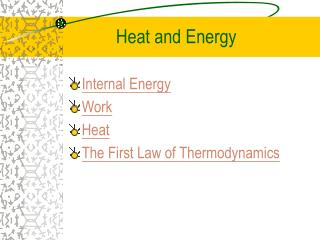DownloadDownload PresentationHeat and Energy

# Heat and Energy

Download Presentation## Heat and Energy

- - - - - - - - - - - - - - - - - - - - - - - - - - - E N D - - - - - - - - - - - - - - - - - - - - - - - - - - -
##### Presentation Transcript

1. Heat and Energy • Internal Energy • Work • Heat • The First Law of Thermodynamics

2. Heat (Q) • Heat is energy transferred as a result of a temperature gradient. • When heat is added • to the system, Q is • positive. When heat is transferred out of the system, Q is negative.

3. Internal Energy (U) - 1 • The internal energy of a system is defined as the sum of the kinetic energies of all its constituent particles, plus the sum of all the potential energies of interaction among these particles.

4. Internal Energy (U) - 2 • The same temperature implies that the average translational kinetic energy is the same.

5. Change in Internal Energy (U) - 1 The change in internal energy: • ΔU can be positive, negative, • or zero for different processes. • The internal energy of a system can be • changed by • doing work, • Heating.

6. F Final state Work (W) • A thermodynamic system can exchange energy with its surroundings by means of heat and work. • A system does work on its surroundings when its volume changes. T = 400 K T = 300 K T = 300 K Heating gas Work done On gas

7. Change in Internal Energy (U) - 2 • The change in internal energy of a system during any thermodynamic process depends only on the initial and final states. • ΔU is independent of path. • For a cyclic process, the total internal energy change must be zero. • The internal energy of an isolated system is constant.

8. The First Law of Thermodynamics (1) • The change in internal energy of a system is equal to the sum of the heat supplied to the system and the work done on the system. • ΔU = ΔQ + ΔW • The 1st law of thermodynamics is a generalization of the principle of conservation of energy to include energy transfer through heat as well as mechanical work.

9. The First Law of Thermodynamics (2) • The heat supplied to a system is equal to the sum of the external work done by the system and the increase in internal energy of the system. • ΔQ = ΔU + ΔW

10. dx pA Work Done by a System during Volume Changes - 1 Consider a gas in a cylinder with a movable piston as shown below. The work done by the system during the small expansion dx is http://www.ux1.eiu.edu/~cfadd/1150/14Thermo/work.html

11. In a finite change of volume from to Work Done by a System during Volume Changes - 2 But A dx = dV ThusdW = p dV If the pressure p remains constant then

12. Work done on/by a system • When work is done on the system, W is negative. (W < 0) • When work is done by the system, W is positive.(W > 0) *For (Q=U+W) only

13. p-V diagram p V 0 Work Done by a System during Volume Changes - 3 Work = Area under the curve

14. Heating a Gas at Constant Volume • Work done by the gas is zero. • U = Q - W = Q

15. Work and Internal energy • DU = Q - W • Expand rubber band:  W < 0, Q = 0DU > 0 temperature increases.